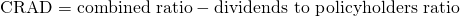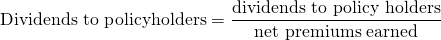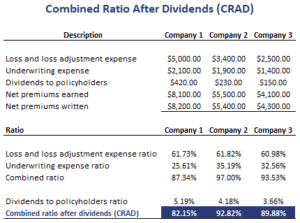# Combined Ratio After Dividends (CRAD)

The Combined Ratio After Dividends (CRAD) is a metric that is used to evaluate the profitability of insurance companies. In particular, the CRAD is often calculated to determine the profitability of Property and Casualty Insurance (P&C) companies. The CRAD ratio starts from the combined ratio. It measures the total efficiency and is more comprehensive than the combined ratio. nevertheless, it is important to be able to calculate the combined ratio first.

On this page, we discuss the combined ratio after dividends formula. We will see that the CRAD is based on two other ratios, which we discuss first. Once we have discussed the combined ratio after dividends definition we turn to an example. An Excel spreadsheet that implements the CRAD example Excel is available at the bottom of the page.

## Combined ratio after dividends formula

The CRAD is defined as followsThus, to calculate the CRAD, we simply need two ratios, the combined ratio and the dividends to policyholders ratio. As for the standard combined ratio, a detailed discussion and Excel spreadsheet can be found under combined ratio.

Let’s turn to the dividends to policyholders (shareholders) ratio. It can be calculated as followsThe dividends to policyholders is a liquidity measure that measures the cash outflow of premium income that goes to the shareholders.

## Combined ratio after dividends ratio example

Let’s have a look at an example. the following table shows how to calculate both the combined ratio and the CRAD for a number of insurance companies.